Next: 5.2.5 Boundary Conditions Up: 5.2 Ray Tracing Previous: 5.2.3 Particle Traversal

## 5.2.4 Algorithmic Complexity

The algorithmic complexity of ray tracing is primarily given by the number of simulated particles which are required to obtain suitable accurate rates, and the effort for calculating a single particle trajectory. The number of simulated particles must scale with the surface area (measured in grid spacings) in order to keep the number of incidences on a disk constant. The surface area, in turn, can be said to be proportional to the number of grid cells which are intersected by the surface. These grid cells can be regarded as the surface discretization elements of the implicit surface representation. Let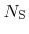be the number of these elements, which allows a comparison with the conventional approach. The number of simulated particles must be of order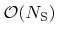.

The effort of tracing a single particle is given by the number of grid cells which must be traversed to find the first surface intersection. The expected number of traversed cells is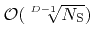. As a consequence, the expected total computational costs scale with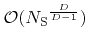. For three dimensions (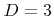) this is already an improved scaling behavior than that of the conventional approach.

The following sections describe how the expected computational effort for finding the first surface intersection point can be further reduced to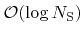. As a consequence, the total effort is equal to. Hence, for large structures with very large, ray tracing is superior to the conventional approach for the calculation of the surface rates and does not require the simplifications of the general model.Next: 5.2.5 Boundary Conditions Up: 5.2 Ray Tracing Previous: 5.2.3 Particle Traversal

Otmar Ertl: Numerical Methods for Topography Simulation